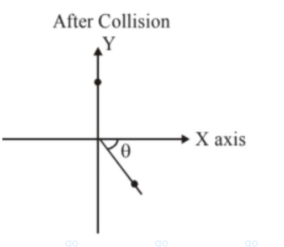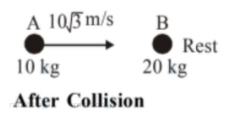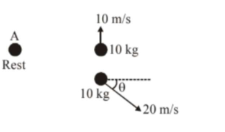# A ball of mass 10 kg moving with a velocityQuestion:

A ball of mass $10 \mathrm{~kg}$ moving with a velocity $10 \sqrt{3} \mathrm{~ms}^{-1}$ along X-axis, hits another ball of mass $20 \mathrm{~kg}$ which is at rest. After collision, the first ball comes to rest and the second one disintegrates into two equal pieces. One of the pieces starts moving along Y-axis at a speed of $10 \mathrm{~m} / \mathrm{s}$. The second piece starts moving at a speed of $20 \mathrm{~m} / \mathrm{s}$ at an angle $\theta$ (degree) with respect to the $X$-axis.

The configuration of pieces after collision is shown in the figure. The value of $\theta$ to the nearest integer is_____Solution:

$(30)$

Before CollisionFrom conservation of momentum along $x$ axis; $\vec{P}_{i}=\vec{P}_{f}$

$10 \times 10 \sqrt{3}=200 \cos \theta$c

$\cos \theta=\frac{\sqrt{3}}{2}$

$\theta=30^{\circ}$